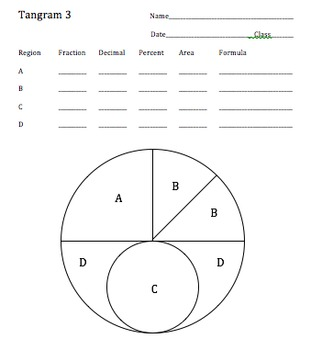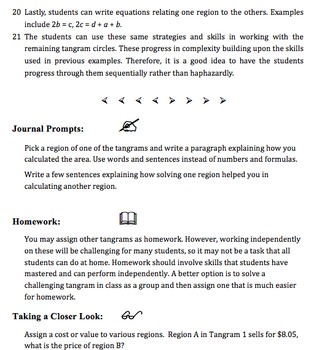# Tangram Circles: Fractions, Geometry, Algebra, and a Generous Helping of PiSubject
Resource Type
File Type

PDF

(6 MB|16 pages)
Standards
• Product Description
• Standards
If your students are working with pi and calculating the areas or circumferences of circles, this activity will give them plenty of practice. Students will use πr2 to calculate the areas of circles and regions within them. Each model progresses in complexity from simple to advanced. This can be used as an individual or team activity with upper middle school and high school students in regular education or more advanced students at lower grade levels. Students can even create their own circular designs using the included template for a culminating project.
Represent proportional relationships by equations. For example, if total cost 𝘵 is proportional to the number 𝘯 of items purchased at a constant price 𝘱, the relationship between the total cost and the number of items can be expressed as 𝘵 = 𝘱𝘯.
Recognize and represent proportional relationships between quantities.
Use square root and cube root symbols to represent solutions to equations of the form 𝘹² = 𝘱 and 𝘹³ = 𝘱, where 𝘱 is a positive rational number. Evaluate square roots of small perfect squares and cube roots of small perfect cubes. Know that √2 is irrational.
Use variables to represent quantities in a real-world or mathematical problem, and construct simple equations and inequalities to solve problems by reasoning about the quantities.
Use rational approximations of irrational numbers to compare the size of irrational numbers, locate them approximately on a number line diagram, and estimate the value of expressions (e.g., π²). For example, by truncating the decimal expansion of √2, show that √2 is between 1 and 2, then between 1.4 and 1.5, and explain how to continue on to get better approximations.
Total Pages
16 pages
Included
Teaching Duration
1 Week
Report this Resource to TpT
Reported resources will be reviewed by our team. Report this resource to let us know if this resource violates TpT’s content guidelines.